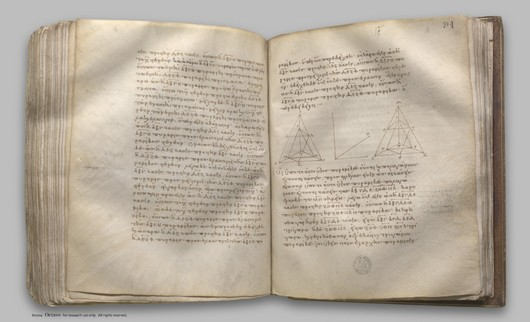## Euclid's Elements, Book XII

 elem.12.1 Similar polygons inscribed in circles are to one another as the squares on the diameters. f. 310 digilib elem.12.2 Circles are to one another as the squares on the diameters. f. 311 digilib elem.12.3 Any pyramid which has a triangular base is divided into two pyramids equal and similar to one another, similar to the whole and having triangular bases, and into two equal prisms; and the two prisms are greater than the half of the whole pyramid. f. 313 digilib elem.12.4 If there be two pyramids of the same height which have triangular bases, and cach of them be divided into two pyramids equal to one another and similar to the whole, and into two equal prisms, then, as the base of the one pyramid is to the base of the other pyramid, so will all the prisms in the one pyramid be to all the prisms, being equal in multitude, in the other pyramid. f. 315 digilib elem.12.5 Pyramids which are of the same height and have triangular bases are to one another as the bases. f. 317 digilib elem.12.6 Pyramids which are of the same height and have polygonal bases are to one another as the bases. f. 318 digilib elem.12.7 Any prism which has a triangular base is divided into three pyramids equal to one another which have triangular bases. f. 319 digilib elem.12.8 Similar pyramids which have triangular bases are in the triplicate ratio of their corresponding sides. f. 320 digilib elem.12.9 In equal pyramids which have triangular bases the bases are reciprocally proportional to the heights; and those pyramids in which the bases are reciprocally proportional to the heights are equal. f. 321 digilib elem.12.10 Any cone is a third part of the cylinder which has the same base with it and equal height. f. 323 digilib elem.12.11 Cones and cylinders which are of the same height are to one another as their bases. f. 325 digilib elem.12.12 Similar cones and cylinders are to one another in the triplicate ratio of the diameters in their bases. f. 327 digilib elem.12.13 If a cylinder be cut by a plane which is parallel to its opposite planes, then, as the cylinder is to the cylinder, so will the axis be to the axis. f. 330 digilib elem.12.14 Cones and cylinders which are on equal bases are to one another as their heights. f. 331 digilib elem.12.15 In equal cones and cylinders the bases are reciprocally proportional to the heights; and those cones and cylinders in which the bases are reciprocally proportional to the heights are equal. f. 331 digilib elem.12.16 Given two circles about the same centre, to inscribe in the greater circle an equilateral polygon with an even number of sides which does not touch the lesser circle. f. 333 digilib elem.12.17 Given two spheres about the same centre, to inscribe in the greater sphere a polyhedral solid which does not touch the lesser sphere at its surface. f. 333 digilib elem.12.18 Spheres are to one another in the triplicate ratio of their respective diameters. f. 337 digilib

Clay Mathematics Institute Historical Archive

Published May 8, 2008. Copyright 2008, Clay Mathematics InstituteProposition 12.6WWW claymath.org

You may also enter Greek text in the search box, e.g. cut and paste from the Greek text on this site.